Next: 5.1.2 Factor Extraction Up: 5.1 Statistical Analysis of Previous: 5.1 Statistical Analysis of

## 5.1.1 Mathematical Background and Application

A wafer map can be formalized by looking at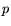different random variables X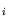which form p-dimen-
sional random vector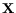=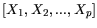. Every component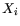can take values n values, where n is equal to the overall number of cells. The mean values of the components are given by: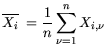(5.1)

while the standard deviations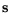=,, read: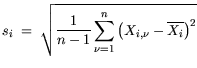(5.2)

Fig. 5.2 shows the results of a DC-RF wafer map of a 4 inch GaAs PHEMT wafer in order to demonstrate the method. Typical DC and small-signal quantities are used that define the vector.The covariance matrix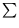with the components: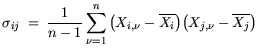(5.3)

and the correlation matrix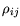:(5.4)

are normally not considered for analysis. Table C.1 in Appendix C shows the correlation matrix of the example data set shown in Fig. 5.2. Measures are taken to test whether the chosen variables in the wafer map are suitable for factor analysis, as described in [22,53]. A significance test is performed to investigate the error probability, whether the variables are uncorrelated. The significance is related to high values of the correlation itself, as can be seen in Table C.1. Only for correlation coefficients close to unity (i.e.,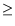0.7) there is a statistically significant probability of correlation, which is not accidental. Further tests can be performed, such as the Barlett test of spericity , which in our example confirms the overall correlation of the data set, so that further analysis is relevant. Third, the so-called anti-image of the covariance and correlation matrix is calculated [22,53]. This assumes that in general the correlation coefficients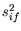are composed as given in (5.5).(5.5)are the observed correlation coefficients and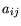the so called partial correlation coefficients. The anti-image controls how much of the observed variance can be explained by a multiple regression analysis within the set of observed variables. The partial correlation coefficientsin (5.5) represent how much of the variance is independent of the other variables in the data set, i.e., dependent on variables not contained in the data set. For the computation, see . This is necessary, since PCA assumes that common factors are the source of variance for the variables Xunder investigation. Another test for the data can be performed, which requires that less than 25% of the non-diagonal elements in the anti-image covariance matrix must be different form zero i.e. numerically,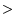0.09. The example passes also this test, since none of the components is0.09. The two matrices are shown in Table C.2 in Appendix C.

From the anti-image correlation matrix the Kaiser-Meyer-Olkin ( KMO) criterion  can be obtained:

 KMO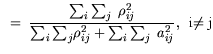(5.6)

whereare the observed correlation coefficients andagain the partial correlation coefficients, i.e., contributions outside of the data set, as separated in (5.5).

An overall KMO criterion close to unity means that the data set is suitable for factor analysis.

For analysis of the contribution to (5.6), a measure of sampling adequacy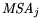can be determined as: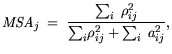i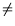j (5.7)

This allows to analyze single variables for their usefulness in the factor analysis. For the whole example the KMO reveals an average of 0.64, which is considered between mediocre and middling on a scale between 0 and 1 established by Kaiser . The variables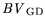,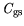,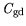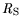,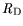, and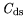have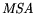values of0.7 and are therefore further used for analysis. The rest of the variables must be discarded as statistically insignificant. Based on the significance information and thethe whole procedure is repeated with the six elements mentioned. The overall KMO criterion with the new reduced dataset reads KMO= 0.73, which is middling according to Kaiser .Next: 5.1.2 Factor Extraction Up: 5.1 Statistical Analysis of Previous: 5.1 Statistical Analysis of
Quay
2001-12-21